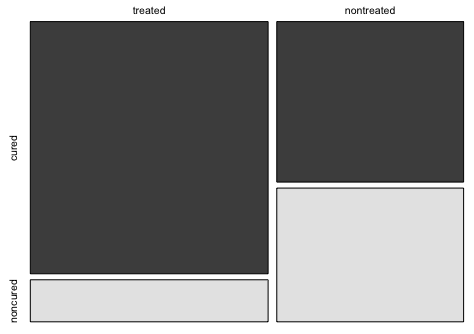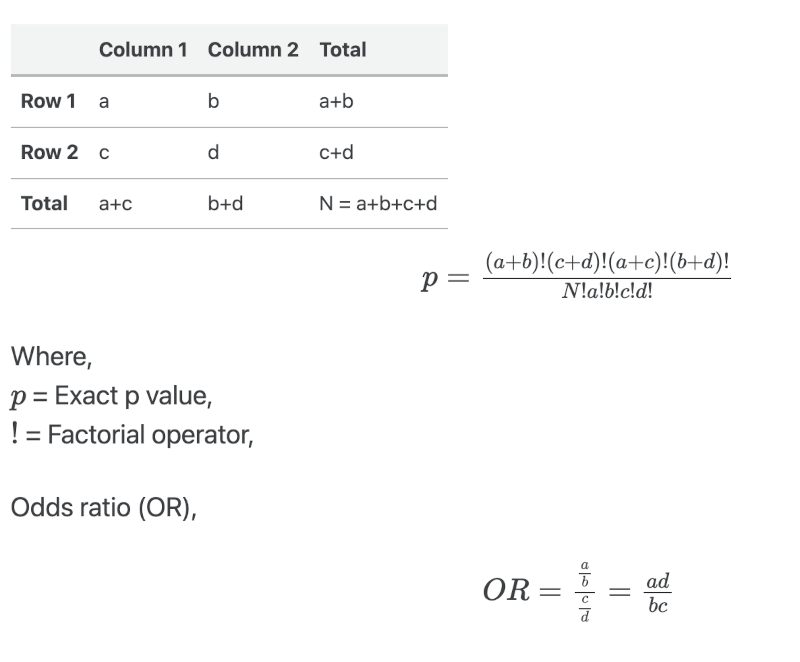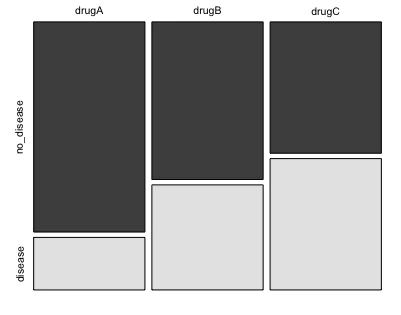# Fisher’s exact test of independence in R [with post-hoc test]## What is Fisher’s exact test

• Fisher’s exact test is a non-parametric method for comparing the proportion of categories in two different independent groups (categorical [nominal] variables) in a contingency table. The categorical variables should be measured dichotomously for 2x2 contingency table (e.g., male/female, treated/no treated, cured/no cured, etc.,). Fisher’s exact test is proposed by Ronald A. Fisher in 1934.
• Unlike the chi-square test, the Fisher’s exact test is an exact test (returns exact p value) and can be applied on smaller sample sizes (<1000). This test is an alternative to the chi-square test, especially when the frequency count is < 5 for more than 20% of cells. If you have larger sample size, it is better to use chi-square test.
• In the Fisher’s exact test, the probability of getting results (observed frequencies) is directly calculated from hypergeometric distribution and not from using any test statistics.
• Fisher’s exact test is most commonly used to analyze a 2x2 contingency table, but it can also be used to analyze data from a larger contingency table. Fisher’s exact test is computationally intensive for larger contingency tables and may not be available in all statistical software.

## Fisher’s exact test assumptions

• The two variables are categorical (nominal) and data is randomly sampled
• The levels of variables are mutually exclusive
• Observations should be independent of each other
• Observation data should be frequency counts and not percentages, proportions or transformed data

## Fisher’s exact test hypotheses

• Null hypothesis: The two categorical variables are independent (no association between the two variables)
• Alternative hypothesis: The two categorical variables are dependent (there is an association between the two variables)

Fisher’s exact test uses the hypergeometric distribution to assess the null hypothesis. Both one and two-tailed hypotheses can be tested using the Fisher’s exact test.

### Fisher’s exact test and odds ratio formula

Suppose, we have the following 2 ✕ 2 contingency table,### Fisher’s exact test for 2x2 contingency table in R

Suppose, there are two categorical variables with binary outcome viz. treatments (treated and nontreated) and treatment outcomes (cured and noncured). In this dataset, we need to test if there is an association between treatments and treatment outcomes.

Check how to perform Fisher’s exact test in Python

### Load and visualize the dataset

Create a Data Frame,

# create a dataframe
df <- data.frame("cured" = c(60, 30), "noncured" = c(10, 25), row.names = c("treated", "nontreated"))
df
# output
cured noncured
treated       60       10
nontreated    30       25


Visualize the dataset,

mosaicplot(df, color = TRUE)### Perform fisher’s exact test

library(stats)
fisher.test(df)
# output

Fisher Exact Test for Count Data

data:  df
p-value = 0.0002357
alternative hypothesis: true odds ratio is not equal to 1
95 percent confidence interval:
1.983312 13.107997
sample estimates:
odds ratio
4.930093


Note: The odds ratio is calculated based on the conditional maximum likelihood estimation (MLE) rather than the sample odds ratio

Check online calculator for Fisher’s exact test

### Interpretation

As the p value (two-tailed) obtained from Fisher’s exact test is significant [p = 0.00023, Odds ratio = 4.93, 95% CI = 1.98-13.10], we reject the null hypothesis (p < 0.05) and conclude that there is a strong association between the two categorical independent variables (treatment and treatment outcomes)

The odds ratio (OR) can be used as an effect size for understanding the treatment effect and decision-making. The odds ratio indicates that the odds of getting cured while on treatment is 4.93 times that of not getting cured. In other words, the person getting treatment is more likely get cured than the person not getting treatment.

### Fisher’s exact test for 3x2 contingency table

Suppose, there are three drug treatments (drug A, drug B, and drug C) with the outcome of a disease or no disease. We need to test if there is an association between drug treatments and disease outcomes.

no disease disease
drug A 40 10
drug B 10 40
drug C 25 25

### Load and visualize the dataset

Create a Data Frame,

# create a dataframe
df <- data.frame("no_disease" = c(40, 30, 25), "disease" = c(10, 20, 25),
row.names = c("drugA", "drugB", "drugC"))
df
# output
no_disease disease
drugA         40      10
drugB         30      20
drugC         25      25


Visualize the dataset,

mosaicplot(df, color = TRUE)### Perform Fisher’s exact test for 3x2 table

library(stats)
fisher.test(df)
# output
Fishers Exact Test for Count Data

data:  df
p-value = 0.005304
alternative hypothesis: two.sided


### Interpretation

As the p value (two-tailed) obtained from Fisher’s exact test is significant [p = 0.005304], we reject the null hypothesis (p < 0.05) and conclude that there is a strong association between the drug treatments and disease symptoms.

### post-hoc test for Fisher’s exact test

After a significant association is identified in a higher contingency table, a post-hoc test for Fisher’s exact is performed to identify pairwise differences. Based on the example of 3x2 contingency table, we do not know which drug and disease outcomes have a significant association.

Here you can do pairwise Fisher’s exact test (using pairwise_fisher_test()) as post-hoc test to analyze each combination. As there are multiple hypothesis testing, p values are corrected using Benjamini-Hochberg FDR method for at a 5% cut-off.

library(rstatix)

# output
* <chr>  <chr>  <dbl>   <dbl>   <dbl> <chr>
1 drugA  drugB    100 0.0486  0.0729  ns
2 drugA  drugC    100 0.00305 0.00915 **
3 drugB  drugC    100 0.422   0.422   ns


The post-hoc test for Fisher’s exact test indicates that there is a significant association [adjusted p = 0.00915] of drug A and drug C with the disease outcome. In other words, the subject is more likely will not have a disease if they receive either of the drug (drug A or drug C).

## Enhance your skills with statistical courses using R

### References

If you have any questions, comments, corrections, or recommendations, please email me at reneshbe@gmail.com